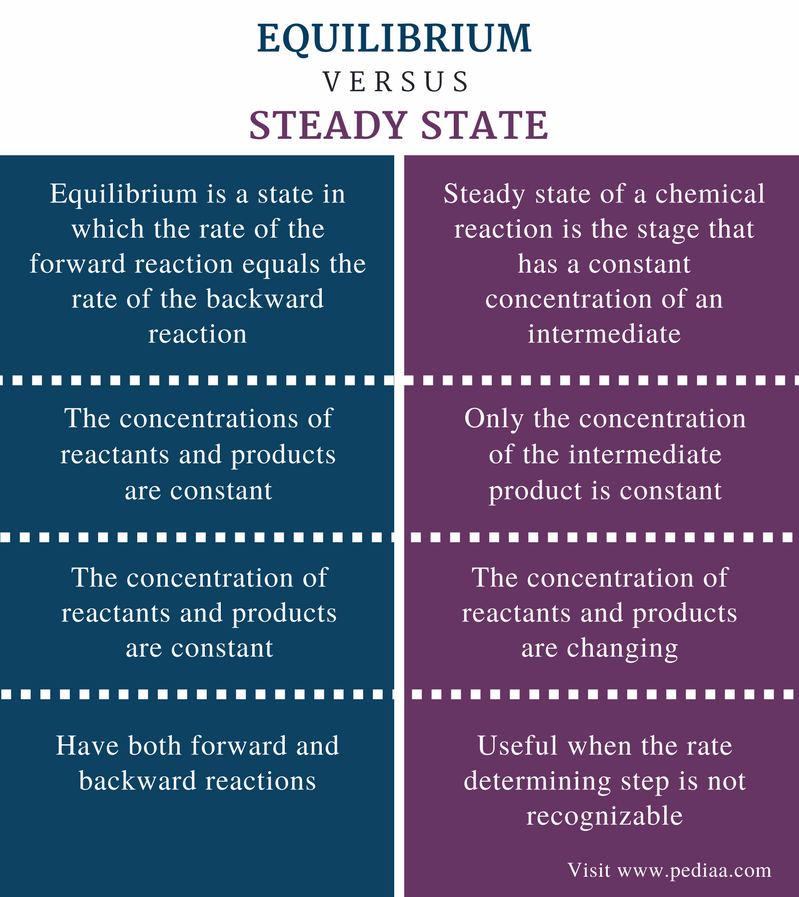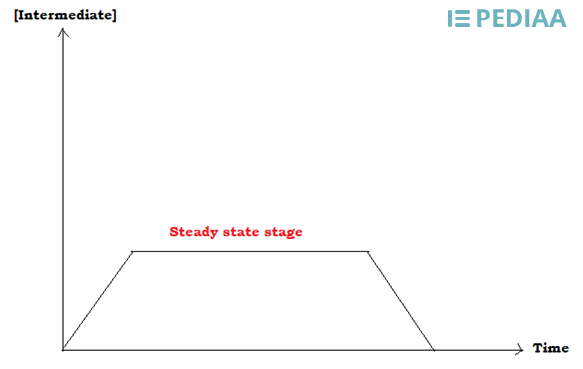# Difference Between Equilibrium and Steady State

## Main Difference – Equilibrium vs Steady State

Equilibrium and steady state are two terms used in physical chemistry regarding chemical reactions that take place in a system. Usually, in a chemical reaction, reactants are turned into products. In some reactions, reactants are completely converted into products but in other reactions, reactants are partially converted into products. Both these terms describe a stage of a particular chemical reaction where the concentrations of the components in the reaction mixture remain constant. But the equilibrium of a reaction is different from the steady state due to several reasons. The main difference between equilibrium and steady state is that equilibrium is a state in which the rate of the forward reaction equals the rate of the backward reaction whereas steady state is the stage of a chemical reaction that has a constant concentration of an intermediate.

### Key Areas Covered

1. What is Equilibrium
– Definition, Principle, Factors Affecting the Equilibrium
– Definition, Principle, Factors Affecting the Steady State
3. What is the Difference Between Equilibrium and Steady State
– Comparison of Key Differences

Key Terms: Equilibrium, Equilibrium Constant, Le Châtelier’s Principle, Products, Reactants, Reaction Rate, Steady State## What is Equilibrium

Equilibrium is a state in which the rate of the forward reaction equals the rate of the backward reaction. Although some chemical reactions reach completion, some other reactions do not completely occur. For example, weak acids and weak bases in aqueous solutions partially dissociate into ions. Then, we can observe there are ions as well as molecules in that solution. Thus, it can be said that there is an equilibrium between molecules and ions (ex: acid and its conjugated base). This happens because the rate of the dissociation of the acid or base is equal to the rate of the formation of acid or base from its ions.

When a reaction mixture is in equilibrium, there is no net change in concentrations of reactants and products. Let us consider an example in order to understand this concept.Figure 1: The equilibrium between acetic acid and its conjugated base

The above image shows equilibrium between acetic acid and its conjugated base. Here, the forward reaction is the dissociation of the acetic acid molecule whereas the backward reaction is the formation of acetic acid molecules.  In order to understand the behavior of an equilibrium system, we can use the Le Châtelier’s principle.

According to the Le Châtelier’s principle, when the equilibrium of a system is disturbed, it tends to obtain an equilibrium state again by changing some of its conditions. In other words, the system tends to readjust itself if the equilibrium is disturbed.

For example, in the above equilibrium, if we add more acetic acid to the solution then the amount of acetic acid is increased in that system. Then in order to obtain the equilibrium, some acetic acid molecules will dissociate, forming the conjugated base and the system will obtain the equilibrium again. In other words, the forward reaction will take place in order to readjust the system.

For systems with an equilibrium, we can define an equilibrium constant. This constant depends on changes in temperature of that system. At a constant temperature, the equilibrium constant always has a fixed value for a certain reaction mixture.

Steady state of a chemical reaction is the stage that has a constant concentration of an intermediate. If a certain chemical reaction occurs through several steps (elementary steps), the rate of the reaction will be determined by the rate-determining step. It is the slowest step among others. Then the rate of the reaction is given regarding this slowest step. But when the reaction steps are not recognizable, the slowest step cannot be recognized in order to determine the rate of the reaction. At such situations, we can consider the intermediate product that has a constant concentration for a short time.Elementary steps of the reaction form intermediate molecules. Intermediates are molecules that are not either reactants or products but are molecules formed during the progression of a chemical reaction. When the slowest step is not recognizable, we can use the concentration of the intermediate for the calculation of the rate of the reaction. This short-lived intermediate is formed in the steady state of the reaction.

## Difference Between Equilibrium and Steady State

### Definition

Equilibrium: Equilibrium is a state in which the rate of the forward reaction equals the rate of the backward reaction.

Steady State: Steady state of a chemical reaction is the stage that has a constant concentration of an intermediate.

### Concentrations

Equilibrium:  In an equilibrium, the concentrations of reactants and products are constant.

Steady State: In steady state, only the concentration of the intermediate product is constant.

### Reactants and Products

Equilibrium:  In equilibrium, the concentration of reactants and products are constant.

Steady State: In steady state, the concentration of reactants and products are changing.

### Reaction Type

Equilibrium:  Equilibriums have both forward and backward reactions.

Steady State:  Steady state is useful when the rate determining step is not recognizable.

### Conclusion

The terms equilibrium and steady state are useful in predicting the rate of a chemical reaction. Although the applications of these terms are different, both equilibrium and steady state explain the behavior of a reaction mixture. The main difference between equilibrium and steady state is that equilibrium is a state in which the rate of the forward reaction equals the rate of the backward reaction whereas steady state is the stage of a chemical reaction that has a constant concentration of an intermediate.

##### References:

1. “Steady-State Approximation.” Chemistry LibreTexts, Libretexts, 20 Apr. 2016, Available here. Accessed 2 Oct. 2017.
2. “Principles of Chemical Equilibrium.” Chemistry LibreTexts, Libretexts, 21 July 2016, Available here. Accessed 2 Oct. 2017.

##### Image Courtesy:

1. “Acetic-acid-dissociation-2D” By Ben Mills – Own work (Public Domain) via Commons Wikimedia